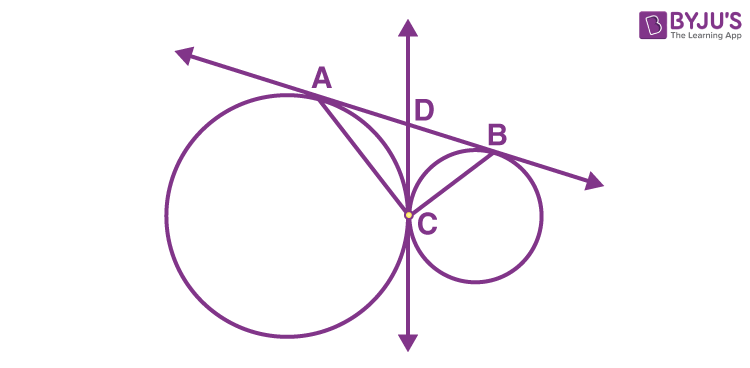# AB and CD are two common tangents to circles which touch each other at C. If D lies on AB such that CD = 4 cm, then AB is equal to (A) 4 cm (B) 6 cm (C) 8 cm (D) 12 cmGiven

CD = 4 cm

We know that tangent drawn from an exterior point are equal

Hence,

From the figure,

CD = AD = 4 cm

CD = BD = 4 cm

Now,

AB = 4 + 4

AB = 8 cm

Therefore, the correct option is (C)(0)(0)